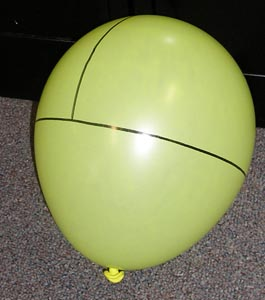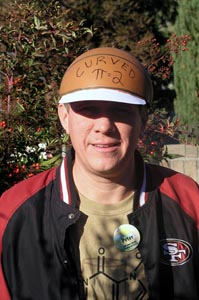Curved Pi

Introduction

The ratio of the circumference of a circle to its diameter in Euclidean space is pi. This ratio takes on different values in curved space. To experience this, measure "curved pi" on the surface of a balloon.A balloon with a circle drawn around its equator and a diameter of the circle drawn through the pole.

Material

• A spherical balloon
• a tape measure
• a marker that will write on the balloon

To Do and Notice

Blow up a balloon.

Consider the inflation nipple as the north pole, draw a line around the equator of the balloon.

Consider that you are an ant on the surface of the balloon. All you know is the surface of the balloon you cannot even conceive of the three dimensional space inside the balloon.

You want to measure the ratio of the circumference of a circle drawn on the balloon to its diameter.

Measure the circumference of the equatorial circle using a tape measure.

Measure its diameter which passes through the south pole.

Calculate curved pi.

(Optional activity, draw a circle on a flat piece of paper, measure its circumference and its diameter. Calculate the ratio of circumference to diameter. The result will approximate the familiar value of pi, 3.14...)

What's Going On?

On the curved surface of the balloon the ratio of the circumference of a circle to its diameter no longer has the same value as pi in ordinary value in flat space.

So What?

We live in a four dimensional space-time. In this space-time we are like the ant. we cannot conceive of the fourth dimension. However, the presence of mass nearby curves space. Where space is flat or gently curved pi has the usual value. But in the presence of dense matter the value of ratio of the circumference of a circle to its diameter changes.

Going Further

Find objects with rotational symmetry and with different curvatures, funnels, bowls, vases.

Draw a circle on these object then measure the circumference and diameter of the circle and calculate the ratio, compare your result to pi.

Math Root

How does the value of curved pi depend on the radius of the balloon?

How does the value of curved pi depend on the latitude of the circle? Assume the circle follows a parallel of latitude. What is curved pi for a circle at 45 degrees south latitude?

Etc.

Pete in his curved pi hat.Peter Geschke in his curved pi hat.

How does the value of curved pi depend on the radius of the balloon?

Etc.

In his novel "Going Postal" Terry Pratchet has a machine that sorts mail into the past and the future. At the heart of this machine is a blue glow that distorts space and time so that pi equals three exactly.